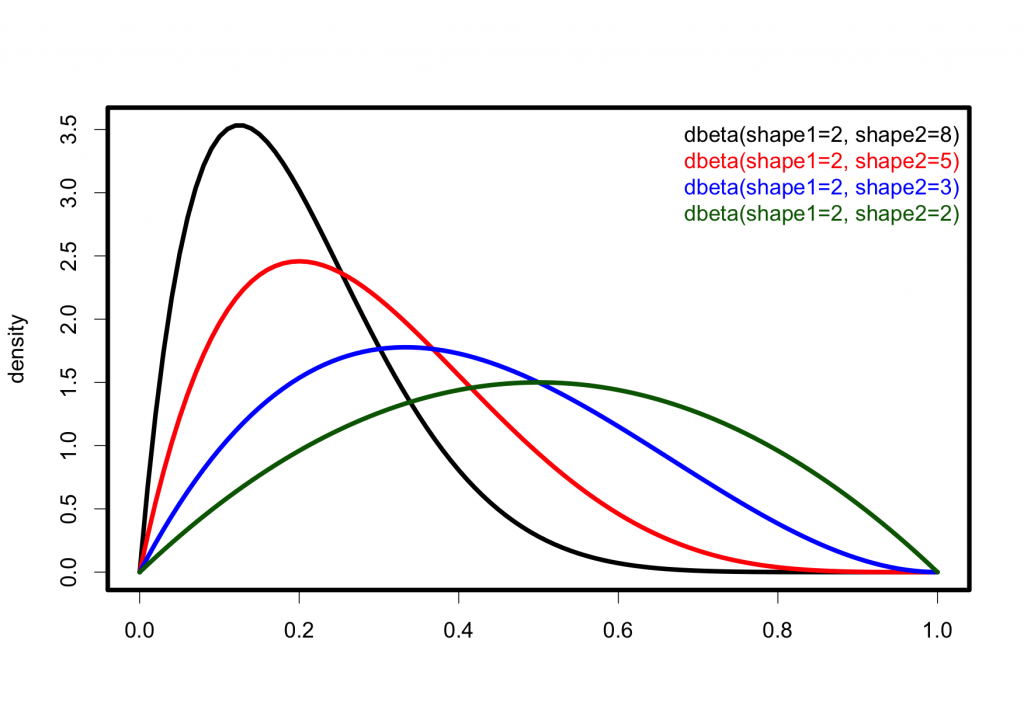# Convenient plotting of distribution shapes in R

I needed to compare the shapes of a few distributions, and therefore wanted an easy way to plot them in R. For example, the standard normal can be plotted quickly enough with `curve(dnorm(x, mean=0, sd=1), from=-3, to=3)`. But when comparing multiple ones, there could be something a bit more handy in terms of keeping track of parameter values for each. So, I ended up writing a little convenience function for that purpose.

As an example, a few beta distributions can be compared with:

```plot_dist(dbeta, c(shape1=2, shape2=8))The code for `plot_dist()` is in this gist.